Next: Dictionary compression Up: Statistical properties of terms Previous: Heaps' law: Estimating the   Contents   Index

## Zipf's law: Modeling the distribution of terms

We also want to understand how terms are distributed across documents. This helps us to characterize the properties of the algorithms for compressing postings lists in Section 5.3 .

A commonly used model of the distribution of terms in a collection is Zipf's law . It states that, if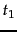is the most common term in the collection,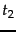is the next most common, and so on, then the collection frequency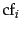of the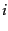th most common term is proportional to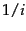: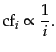(3)

So if the most frequent term occurs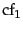times, then the second most frequent term has half as many occurrences, the third most frequent term a third as many occurrences, and so on. The intuition is that frequency decreases very rapidly with rank. Equation 3 is one of the simplest ways of formalizing such a rapid decrease and it has been found to be a reasonably good model.

Equivalently, we can write Zipf's law as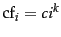or as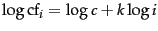where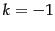and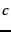is a constant to be defined in Section 5.3.2 . It is therefore a power law with exponent. See Chapter 19 , page 19.2.1 , for another power law, a law characterizing the distribution of links on web pages.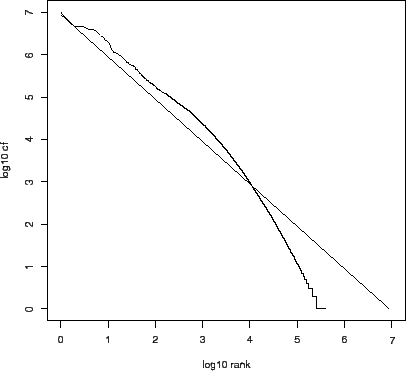Zipf's law for Reuters-RCV1. Frequency is plotted as a function of frequency rank for the terms in the collection. The line is the distribution predicted by Zipf's law (weighted least-squares fit; intercept is 6.95).

The log-log graph in Figure 5.2 plots the collection frequency of a term as a function of its rank for Reuters-RCV1. A line with slope -1, corresponding to the Zipf function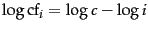, is also shown. The fit of the data to the law is not particularly good, but good enough to serve as a model for term distributions in our calculations in Section 5.3 .

Exercises.

• Assuming one machine word per posting, what is the size of the uncompressed (nonpositional) index for different tokenizations based on Table 5.1 ? How do these numbers compare with Table 5.6 ?Next: Dictionary compression Up: Statistical properties of terms Previous: Heaps' law: Estimating the   Contents   Index